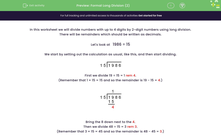# Formal Long Division (2)

In this worksheet, students must divide numbers up to 4 digits by a two-digit number using the formal written method of long division. There may be remainders, which should be written as decimals.Key stage:  KS 2

Curriculum topic:   Number: Addition, Subtraction, Multiplication and Division

Curriculum subtopic:   Divide to Four Digits (Long Division)

Difficulty level:#### Worksheet Overview

In this worksheet we will divide numbers with up to 4 digits by 2-digit numbers using long division.

There will be remainders which should be written as decimals.

Let's look at   1986 ÷ 15

We start by setting out the calculation as usual, like this, and then start dividing.First we divide 19 ÷ 15 = 1 rem 4.

(Remember that 1 × 15 = 15 and so the remainder is 19 - 15 = 4.)Bring the 8 down next to the 4.

Then we divide 48 ÷ 15 = 3 rem 3.

(Remember that 3 × 15 = 45 and so the remainder is 48 - 45 = 3.)Bring the 6 down next to the 3.

Now we divide 36 ÷ 15 = 2 rem 6.

(Remember that 2 × 15 = 30 and so the remainder is 39 - 30 = 9.)The final remainder is 6, but we want to write this as a decimal.

We now change 1986 to 1986.000.

We're not sure how many zeros we will need, but it doesn't matter because

1986 = 1986.0 = 1986.00 = 1986.000 etc.Bring the new 0 down next to the 6.

Now we can divide 60 ÷ 15 = 4 rem 0.

(Remember that 4 × 15 = 60 and so the remainder is 60 - 60 = 0.)

We must put a decimal point in the answer line above the decimal point of 1986.000.We didn't in fact need the last two zeros, but remember that we didn't know how many we would need.

We have shown that 1986 ÷ 15 = 132.4

### What is EdPlace?

We're your National Curriculum aligned online education content provider helping each child succeed in English, maths and science from year 1 to GCSE. With an EdPlace account you’ll be able to track and measure progress, helping each child achieve their best. We build confidence and attainment by personalising each child’s learning at a level that suits them.

Get started